# Control Structure: Guide To Learning Loops In PHP

In our previous guide, we've seen conditional statements in PHP that lets us alter the program flow base on conditional choices, in this guide, we would look into the different types of loops in PHP. Loops give us the ability to write code that would execute more than once without the need of writing the code over and over again.

Imagine having to edit 10,000 lines of text within a CSV file, this would be a shit load of work if done manually, which is where looping comes in. When a task or a series of tasks needs to be repeated, it can be put inside a loop.

We would look into various types of loops, e.g While Loops, For Loops, For Each Loops, and a couple of ways to enhance the Loops using break and continue statement, let's start with...

## While Loops

A while loops while the condition is true, the code executes once
and then it loops back to the start to execute again. Each time the code executes, it might change the values and variables each time it goes through the loop.

The syntax of a while loops is as follows:

``````while (expression){
statement;
}``````

This is really easy to understand, if you think of them in the following way: While the expression evaluates to True, keep executing or looping through the statements.

Let's see an example:

``````<?php

\$count = 10;
\$n = 0;
while (\$count >= 0) {
echo ++\$n . " " . " Welcome To Devsrealm\n";
\$count--;
}

#
# Output
#
1  Welcome To Devsrealm
2  Welcome To Devsrealm
3  Welcome To Devsrealm
4  Welcome To Devsrealm
5  Welcome To Devsrealm
6  Welcome To Devsrealm
7  Welcome To Devsrealm
8  Welcome To Devsrealm
9  Welcome To Devsrealm
10  Welcome To Devsrealm
11  Welcome To Devsrealm``````

Note: It prints "Welcome To Devsrealm" 11 times because you are saying while the count is greater than 0 or equal to 0, so, when the count gets to 0, it still prints the statement before breaking out of the loop. If you want to print the "Welcome To Devsrealm" 10 times, then you need to do: while (\$count >= 1)

The above example prints "Welcome To Devsrealm" 11 times, it does the following:

• We set an initial value of where the count should begin, in this case, we want the count to begin at 10; \$count =10
• We then say, “while the count is greater than or equals to 0, do the next step, for the first run, it is true, we have 10, so…
• It prints "Welcome To Devsrealm"
• count-- reduces the count by 1, you can also write it as count=count – 1
• As soon as the count reaches -1, it skips the while loop altogether

Here is an illustration of how it works: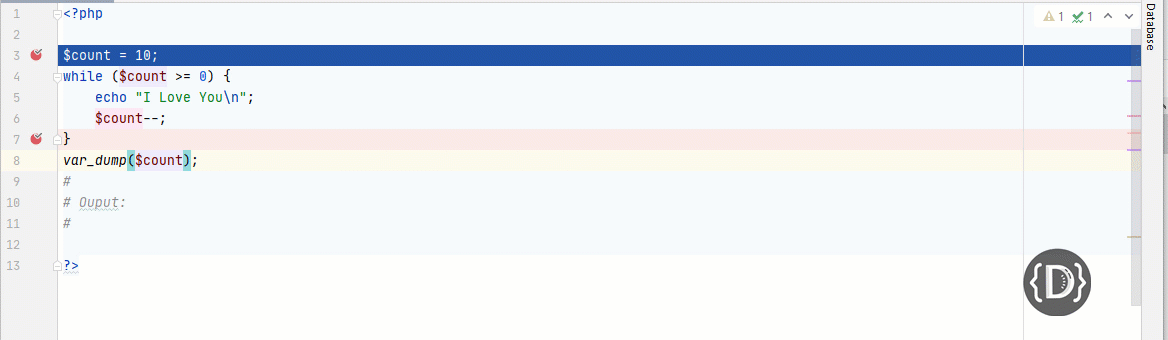As you can see in the illustration above, it keeps reducing the value of \$count variable by one, it then checks if the value in the \$count is greater than or equals to zero, if no, it executes the echo statement, and reduces the count by 1 on every run until it reaches -1, it then jumps out.

One thing you need to note is that, the loop needs to have condition, and an expression that will allow it to execute, and secondly, something about the condition has to change during the course of the loop, if anything doesn't change, it would be an infinite loop, which is not something you would want.

For example, if we remove the \$count--, you would get an infinite loop, here is an illustration: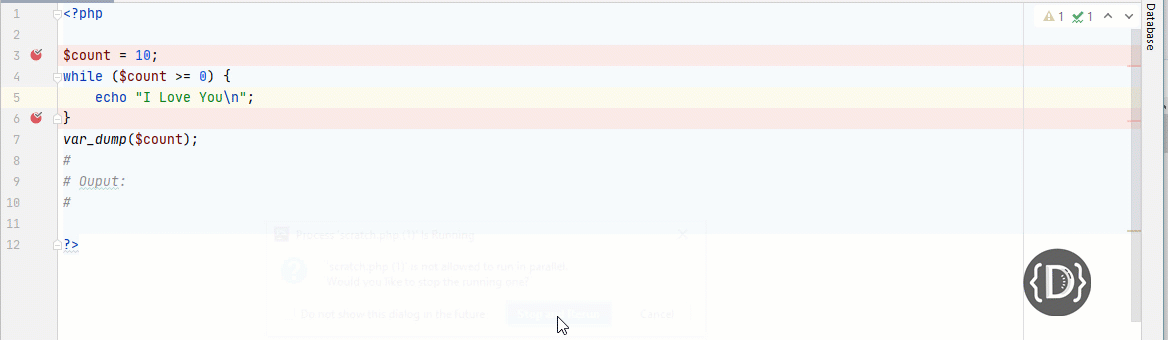As you can see in the image, the loop is stuck, it would print "I Love You" forever or until PHP times out, and this is because 10 is always greater than zero(0), so, something has to change or you need to somehow reduce the value until you get out of the loop, you can't just keep on going forever.

Here is another example that prints from 0 to 10:

``````<?php

\$count = 0;
while (\$count <= 10) {
echo "{\$count}, ";
\$count++;
}

#
# Output:
#

0, 1, 2, 3, 4, 5, 6, 7, 8, 9, 10,``````

So, again, we did the following:

• We set an initial value of where the count should begin, in this case, we want the count to begin at 0; \$count =0
• We then say, “while the count is lesser than or equals to 0, do the next step, the first run is true, we have 0, so…
• It prints `0, `
• count++ increases the count by 1, you can also write it as count=count + 1
• As soon as the count reaches 11, it skips the while loop altogether

Here is an illustration of how it works: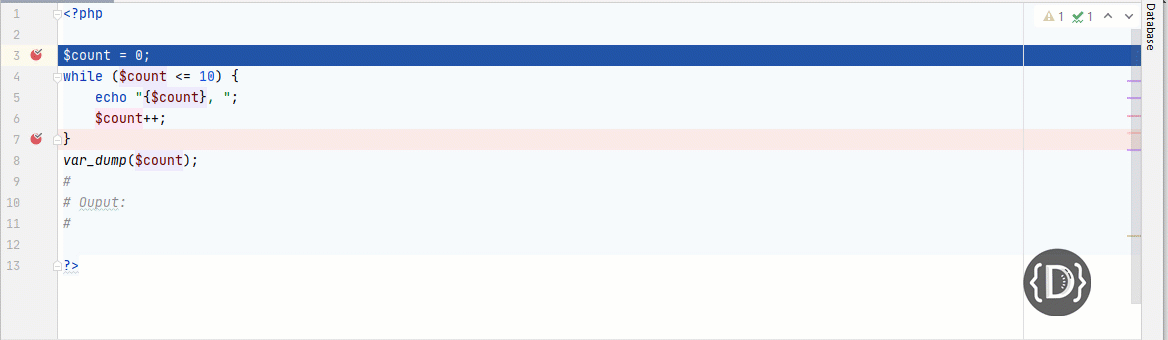As you can see in the illustration above, it keeps incrementing the value of \$count variable by one, it then checks if the value in the \$count is lesser than or equals to 10, if false, it executes the echo statement, and increments the count by 1 on every run until it reaches 11, it then jumps out.

I guess this clarifies while loops in PHP.

## For Loops

A for loops is similar to a while loop, in that it loops while the condition is true,  the code executes once, and then it loops back to the start to execute again. The syntax of for loops is a bit more classical than while loop, here is the syntax:

``````for (expr1; expr2; expr3) {
statement;
}``````

Instead of setting the initial value outside of the loop, like the way we did it in the while loop, you put that in expression 1, so, it would be executed the first time only, expression 2 is what we are evaluation or what we are testing, and expression 3 is going to be executed at the end of every loop; an example is the count++ we used in the while loop.

The following is an example of for loop:

``````<?php

for (\$count = 0; \$count <= 10; \$count++) {
echo "{\$count}, ";
}
#
# Ouput:
#
0, 1, 2, 3, 4, 5, 6, 7, 8, 9, 10,``````

As you can see it is exactly the same, for loop just gives us a better structure of how everything relates.

Note: Make sure you are not adding a semi-colon to the third expression, here:  `\$count++`

So, feel free to use whatever loops feel right to you.

## Foreach Loops

Foreach loops are different from a while and for loops, a for each loop lets you iterate (perform again) over a series of words within a string or a list of items.

So, it is going to take an array, and then loop through each item of the array until it gets to the end.

You might need to refresh your understanding of arrays, this would make learning foreach a breeze.

When we are using while loops or for loops, we know when to quit or keep looping by testing if a condition has been met, on the other hand, Foreach loops knows when to quit by whether items are left in the list or the array to loop over.

Note: foreach works only on arrays and objects, and will issue an error when you try to use it on a variable with a different data type or an uninitialized variable.

The syntax of foreach loops is as follows:

``````foreach (\$array as \$value) {
statement;
}``````

As you can see the syntax is a bit different from the loops we've seen so far, the foreach loops take an \$array, which is what you would be looping through (it doesn't have to be called \$array, you can call it \$list or whatever you feel makes the most sense), we have a special keyword "as" and the \$value (it doesn't have to be called \$value) is going to be assigned an item as it loops through the items in the \$array.

Note: The \$array should be something that exists beforehand, you can't just loop through a list that does not exist, on the other hand, the \$value doesn't exist, it is just a placeholder for holding the value.

Another thing to note is that when using while or for loops, we create a couple of conditions that tells the loop when to start, incrementing, and when to stop, when using foreach loop, you don't need that, it knows when to start the loop, when to go to the next line, and when to end the loop, well, that is because the condition for exiting is when it gets to the end of the array.

Consider the following example:

``````<?php

\$list = array(1, 2, 3, 4, 5, 6, 7, 8, 9, 10);

foreach (\$list as \$number) {
echo "Number: {\$number}\n";
}

#
# Output:
#

Number: 1
Number: 2
Number: 3
Number: 4
Number: 5
Number: 6
Number: 7
Number: 8
Number: 9
Number: 10``````

We created an array that stores numbers 1 -10 in the `\$list`variable, this is going to store the list of what we are going to loop through. So, we started with the foreach keyword, and we then declare `\$number`to be the variable that will take different values contained in `\$list array`, what we are basically doing is reading from the list on the left to populate the variable parameter (\$number) on the right.

Imagine, you have the item listed in the following order:

• 1
• 2
• 3
• ....
• 10

The \$list contains the array items, so the first time the loops goes, it would assign the first item, in this case, `1`it would store it in the \$number variable, in the second line, we are echoing the output of the \$number variable, it then goes over to pick number `2`until there is nothing left in the array. That is the idea of a foreach loop, as long as there are items to be processed in the list, the loop will execute until the list is exhausted.

Here is an illustration: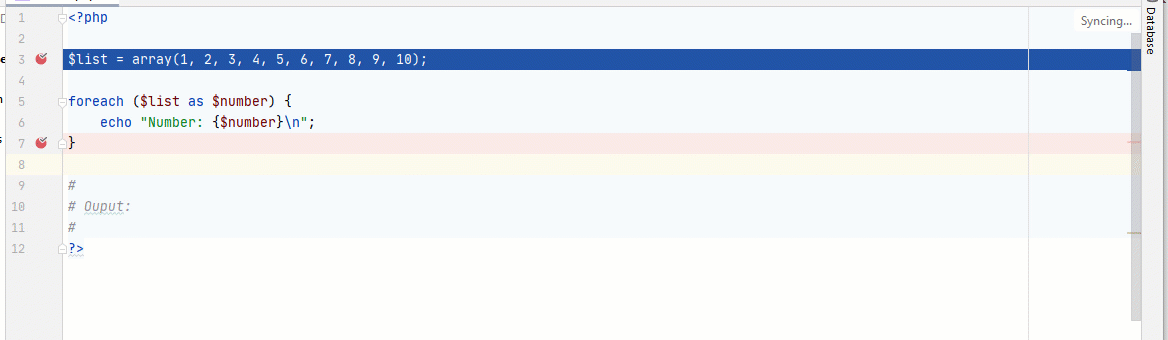The  tells us we have 10 items in the array, and as you can the \$number variable keeps picking the item one after the order, and once it gets to the end of the list, it stops the loop.

Imagine you have a list of a users last 20 orders that you want to output to them, you can easily use foreach loop to get it nicely done.

If you have taken a sneak peek at the array I linked to above, you might have seen the section - "Associative Arrays", so, how do we make foreach loop work with an associative array?

Associative arrays associate items with a label, so whenever we are querying the array, we use the label. The syntax to use them with the foreach is as follows:

``````foreach (\$array as \$key => \$value) {
statement
}``````

We are going to have `\$array`, keyword `as`and then we are going to have two variables, one for the \$key and the other for the \$value, you don't have to call then \$key and \$value though, call them anything that makes the most sense to you.

Let's see an example:

``````<?php

\$userInfo = array(
"first_name"    => "Pascal",
"last_name"     => "Faruq",
);

foreach (\$userInfo as \$attribute => \$data) {
\$attr_format = ucwords(str_replace("_", " ", \$attribute));
echo "{\$attr_format}: {\$data}
\n";
}

#
# Output:
#

First Name: Pascal
Last Name: Faruq

Just like before, we loop through the array, but in this case, we have the key and value (\$attribute => \$data), on the next line, we cleaned up the \$attribute, we simply replaced the underscore (_) with a space, then capitalize the first word, that is the function of the ucwords. Lastly, we echoed the data.

So, whenever you are wanting with an array, you might want to consider using foreach loops first before considering any other kind of loops, foreach is made exclusively for arrays and objects.

## Controlling the Loop with Break and Continue

What if at some point, we need to exit the loop prematurely or perhaps exclude certain items from processing, For this scenario, we have loop control keywords, such as break and continue.

The break keyword is used to break out of a loop, be it a for or while loop, the continue keyword is used to end the current iteration in a loop, but continue with the next iteration.

Let's see an example that creates an odd number with for loops and the continue statement:

``````<?php

for (\$i=0; \$i <= 10; \$i++) {
if (\$i % 2 == 0) { continue; }
echo \$i . ", ";
}

#
# Output:
#

1, 3, 5, 7, 9,``````

An odd number is an integer that can't be divided by 2, Odd numbers when divided by 2, leave a remainder other than zero(0).  Using that definition we can write a program that does that, all we need to do is create a condition that terminates the current iteration whenever the current number returns zero when divided by 2, which is why we have:

`if (\$i % 2 == 0) { continue; }`

For example, the first count is zero (0), and when divided by 2 returns zero(0), the loop terminates the current iteration and goes to the next digit, which is 1, so, when you have 1 divide 2, it returns a reminder other than zero, so, it continues the operation, so, that is the idea of how it works.

You can do the same for an even number, so, you just check if the reminder is not == 0, if the reminder is not == 0, it means it is an odd number, we then use the continue statement to skip it:

``````<?php

for (\$i=0; \$i <= 10; \$i++) {
if (\$i % 2 !== 0) { continue; }
echo \$i . ", ";
}

#
# Ouput:
#
0, 2, 4, 6, 8, 10,``````

You can achieve the same stuff with a while loop:

Disclaimer: There is a problem with the below code, I'll explain...

``````<?php

\$i = 0;
while (\$i <= 10) {
if (\$i % 2 == 0) {
continue;
}
echo \$i . ", ";
\$count++;
}``````

Can you spot the issue?  Well, here is an illustration: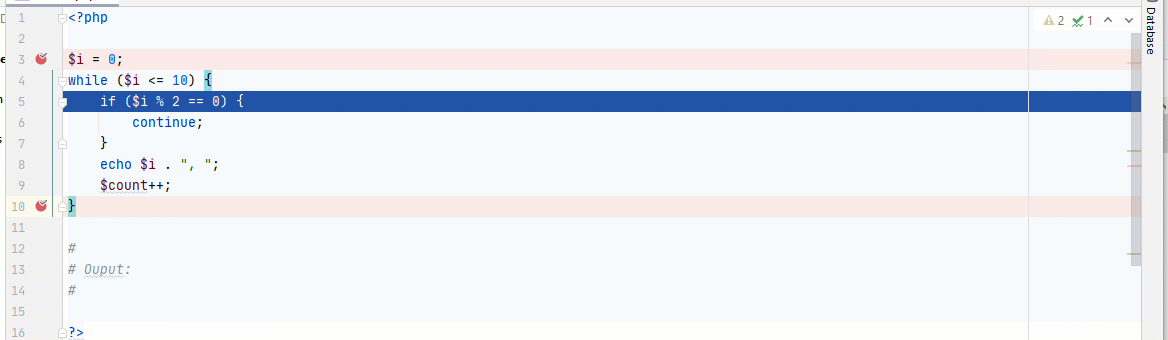You can see we have an infinite operation, which is not what you would want, what happens is as soon as you get to the modulus condition, the loop got stuck. We said if \$i (which is 0) divide by 2 returns zero(0), it should continue, and that goes back to the top of the loop, here:

`while (\$i <= 10)`

and it evaluates \$i is less than or equal to 10, and \$i is zero, so, it goes back to the modulus condition and keeps looping around forever, we are not incrementing it, to fix that, you do:

``````<?php

\$i = 0;
while (\$i <= 10) {
if (\$i % 2 == 0) {
\$i++;
continue;
}
echo \$i . ", ";
\$i++;
}

#
# Output:
#

1, 3, 5, 7, 9,``````

Now, before we continue the modulus condition, we increment it, it is as simple as that. This doesn't happen in for loop because it does the incrementation at the beginning stage of the loop, so, that is the reason why some people prefer it.

Let's get into the break statement, it breaks out of the loop entirely, consider the following example:

``````<?php

for (\$i=0; \$i <= 10; \$i++) {
if (\$i == 5) {
break;
}
echo \$i . ", ";
}

#
# Ouput:
#

0, 1, 2, 3, 4,``````

As soon, as the \$i variable gets to 5, it breaks out of the loop entirely unlike a continue statement that would cancel the current iteration, and move on with the next one.

This conclude our guide on loop in PHP, hope you find it helpful

## Related Post(s)

• ### Guide To Laravel - Model and Database Migrations

I don't know if you have read my guide on Creating a Tiny PHP MVC Framework From Scratch where we create a tiny MVC framework in the hope of understanding the concepts of how major frameworks imple

• ### Building Dependency Injection and The Container from Scratch in PHP

In this guide, you'll learn about dependency injection and a way to build a simple DIC (Dependency Injection Container) in PHP using PSR-11 from scratch. First, What is an Ordinary Dependency? This

• ### Creating a Tiny PHP MVC Framework From Scratch

In this guide, we would go over creating a tiny PHP MVC Framework, this would sharpen your knowledge on how major frameworks (e.g Codeigniter or Laravel) works in general. I believe if you can unders

• ### PHP Pluggable and Modular System – Part 2 (Implementation) [Event Dispatcher]

In the first series of this guide, we discussed the theoretical aspect of building a pluggable system in PHP, I wrote a bit of code in that guide plus a couple of stuff you should avoid, you can lear

• ### Best Way To Implement a Non-Breaking Friendly URL In PHP or Laravel or Any Language

I was working on the link structure of my new Laravel app, and out of the blue I said: "What would happen if a user changes the slug of a post?" First Attempt - 301 Redirection The first solution I t

• ### PHP Pluggable and Modular System - Part 1 (Abstract View) [Observable and Mediator Pattern]

If there is one thing that is brutally confusing to me ever since I started coding, it would be a way to not only implement a Modular system but a way to make it extensible (adding and removing plugi